#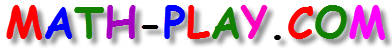The 3rd grade math games on this webpage focus on several important topics such as place value, addition and subtraction of whole numbers and decimals, multiplication and division of whole numbers, concepts of length, perimeter, area, and time, characteristics of geometric figures, as well as collecting, organizing, displaying, and interpreting data.

Learning math has never been so much fun!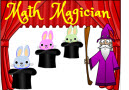Math Magician

Are you a math magician? Make 20 bunnies disappear by solving addition, subtraction, and multiplication problems very quickly.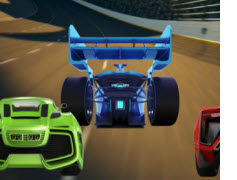Math Racing Game Addition with Regrouping

Be a part of the excitement of playing car racing with this great Math Racing Game Addition with Regrouping. Here you will add numbers correctly to continue in the race to the finish line. Work quickly so you can cross the finish line first.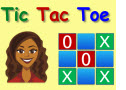Even or Odd?

This is a fun and interactive Tic Tac Toe game about classifying whole numbers as even or odd.Rounding Numbers Pirate Game

Round numbers correctly in this fun online math pirates game to search for the treasure chest.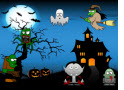3rd Grade Halloween Math Game (New)

Play this spooky Halloween math game and practice your math measurement skills to destroy a lot of monsters. For each correct answer, you will enter a bonus round where you can earn points by smashing monsters. The math problems are about measuring time, volume, and mass.Math Racing Game Number Facts

Enjoy car racing fun with this great Math Racing Game Number Facts. You must solve problems of the four operations to continue in the race to the finish line. Work quickly so you can cross the finish line first.Math Racing Game Multiply within 100

The fun, the excitement, the roar of the engines are all here in this Math Racing Game Multiply within 100. Multiply numbers quickly and accurately in order to get to the checkered flag.Math Racing Game Divide within 100

Hear the roar of the engines, see the hairpin turns as they quickly approach in this Math Racing Game Divide within 100. You must divide numbers quickly to continue in the race to the finish line or your "racecar" could spin-out and your race is done.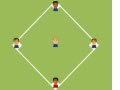Multiplication Facts up to 12 Baseball Game
Have fun practicing your multiplication skills by playing this exciting Baseball Math Multiplication Facts up to 12 Game.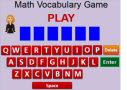3rd Grade Math Vocabulary Game (New)

3rd grade students will have fun identifying important math terms when playing this interactive vocabulary game. For each definition, the students will have only 60 seconds to identify the correct word.3rd Grade Rounding Halloween Math Game (New)

Soar into great math skills by playing this 3rd Grade Rounding Halloween Math Game and get loads of practice rounding numbers to the nearest ten and hundred.Math Magician Division Game

Play this fun and interactive game and make 20 bunnies disappear by quickly matching different division problems with the correct answer.Division up to 100 Baseball Game
Make learning and improving your multiplication skills by playing this exciting Baseball Math Division up to 100 Game.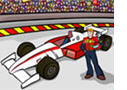Multiplication Game

In this fast-paced car racing game, students will practice multiplication facts to 10 times 10.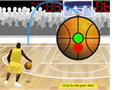Basketball Multiplication Game

In this fun basketball game, young students will have fun multiplying one-digit numbers.Math Magician Rounding Game

Match the rounding problems with the correct solutions on these little bunnies in this fun Math Magician Rounding Game.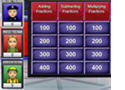Multiplication Jeopardy Game

In this game students will multiply one-, two-, and three-digit numbers by 5, 6, 7, 8, and 9. Kids can play this game alone or in teams.Multiplication as Repeated Addition

In this fast-paced racing game students will use repeated addition to model multiplication problems.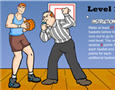Basketball Math - Properties of Multiplication

In this fast-paced math game, students will identify and use the commutative, associative, and identity property of multiplication.Multiplication Basketball Game

In this game students will multiply 2-digit numbers by 1-digit numbers. They can play it alone or in pairs.Counting Coins

In this game students will count various US coins and match the pictures of the coins with the correct amounts.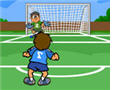Adding Two-Digit Numbers

In this fun soccer game, students will add two-digit numbers to get a chance to kick the ball and score points.Subtracting Two-Digit Numbers

In this interactive soccer game, 2nd grade students will practice adding 2-digit numbers.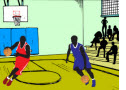Short Division Basketball Game

Third grade students will have fun dividing small numbers when playing this math basketball game.Place Value Game

In this fun place value game, students must pass the ball to the receiver in order to be given the chance to answer a problem and earn points.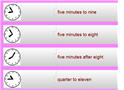Time Matching Game

This game is suitable for 3rd grade students and English language learners of all ages. The object of each problem is to match the analog clocks with the correct phrase.Telling Time Basketball Game

In this interactive basketball game, 3rd grade students will practice telling time from analog clocks to the nearest minutes.Who has? I have!

This is a printable time game that can be used as a classroom activity with elementary students.Math Magician Multiplication Game

Match the multiplication problems with the correct solutions on these little bunnies in this fun Math Magician Multiplication Game.

Return from the 3rd Grade Math Games page to the Elementary Math Games page or to the Math Play homepage.